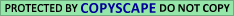## A.2. The Corrected Distance

In the previous section we defined a measure W(w,w') of proximity between two words w and w' -- an inverse measure of the distance between them. We are, however, interested less in the absolute distance between two words than in whether this distance is larger or smaller than "expected." In this section, we define a "relative distance" c (w,w'), which is small when w is "unusually close" to w', and is 1, or almost 1, when w is "unusually far" from w'.

The idea is to use perturbations of the arithmetic progressions that define the notion of an ELS. Specifically, start by fixing a triple (x,y,z) of integers in the range {-2,-1,0,1,2}; there are 125 such triples. Next, rather than looking for ordinary ELS's (n,d,k), look for "(x,y,z)- perturbed ELS's" (n,d,k)(x,y,z), obtained by taking the positions

n, n + d,..., n + (k-4)d, n + (k-3)d + x, n + (k-2)d + x + y, n + (k-1)d +x +y + z

instead of the positions n, n +d, n + 2d,..., n + (k-1)d. Note that in a word of length k, k-2 intervals could be perturbed. However, we preferred to perturb only the three last ones, for technical programming reasons.

The distance between two (x,y,z)-perturbed ELS's (n,d,k)(x,y,z) and (n',d',k')(x,y,z) is defined as the distance between the ordinary (unperturbed) ELS's (n,d,k) and (n',d',k').

We may now calculate the "(x,y,z)-proximity" of two words w and w' in a manner exactly analogous to that used for calculating the "ordinary" proximity W (w,w'). This yields 125 numbers W ((x,y,z) (w,w'), of which W (w,w') = W(0,0,0)(w,w') is one. We are interested in only some of these 125 numbers; namely, those corresponding to triples (x,y,z) for which there actually exist some (x,y,z)-perturbed ELS's in Genesis for w, and some for w' [the other W(x,y,z) (w,w') vanish]. Denote by M(w,w') the set of all such triples, and by m(w,w') the number of its elements.

Suppose (0,0,0) is in M (w,w'), that is, both w and w' actually appear as ordinary ELS's (i.e., with x = y = z = 0) in the text. Denote by v (w,w') the number of triples (x,y,z) in M (w,w') for which W(x,y,z) (w,w') >= W (w,w'). If m (w,w') >= 10 (again, 10 is an arbitrarily selected "moderate" number),

c (w,w') := v (w,w') / m (w,w').

If (0,0,0) is not in M (w,w'), or if m (w,w') < 10 (in which case we consider the accuracy of the method as insufficient), we do not define c (w,w').

In words, the corrected distance c (w,w') is simply the rank order of the proximity W (w,w') among all the "perturbed proximities" W(x,y,z) (w,w'); we normalize it so that the maximum distance is 1. A large corrected distance means that ELS's representing w are far away from those representing w', on a scale determined by how far the perturbed ELS's for w are from those for w'.

Click Here to Go To Appendix A.3.